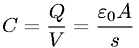Equations > Physics > Electricity and Magnetism > Capacitance of a parallel-plate capacitor

### Capacitance of a parallel-plate capacitorLatex Code:

MathML Code:

 $C=\fracQV=\frac{ϵ}_{0}As$

MathType 5.0: